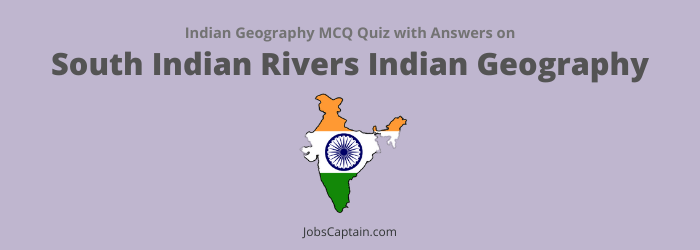# MCQ on South Indian Rivers Set 2: Indian Geography QuizQuestion 1. The correct sequence of the Eastward flowing rivers of the peninsular India from North to South is __________.

(A) Mahanadi, Subarnarekha, Krishna, Godavari, Cauvery, Vagai and Pennar

(B) Mahanadi, Subarnarekha, Godavari, Cauvery, Pennar and Vagai

(C) Subarnarekha, Mahanadi, Krishna, Godavari, Cauvery, Pennar and Vagai

(D) Subarnarekha, Mahanadi, Godavari, Krishna, Pennar, Cauvery and Vagai

(D) Subarnarekha, Mahanadi, Godavari, Krishna, Pennar, Cauvery and Vagai

Question 2. The rivers of South India mainly have which of the following drainage patterns?

(A) Trellis

(B) Dendritic

(C) Intermittent

(B) Dendritic

Question 3. Which one of the following statements is not correct?

(B) Cauvery River rises in Andhra Pradesh

(C) Godavari River rises in Maharashtra

(D) Mahanadi River rises from Chhattisgarh plateau

(B) Cauvery River rises in Andhra Pradesh

(A) Aravali

(B) Eastern Ghats

(C) Amarkantak

(D) Pulamu hills

(C) Amarkantak

Question 5. The river, which differs from others is __________.

(B) Tapti

(C) Indus

Question 6. Which of the following rivers does not make estuary?

(B) Mandavi

(C) Tapti

Question 7. Which river forms its delta in Odisha?

(A) Tapti

(D) Godavari

Question 8. At which one of the following places do two important rivers of India originate while one of them flows towards North and merges with another important river flowing towards Bay of Bengal, the other one flows towards Arabian Sea?

(A) Nasik

(B) Mahabaleshwar

(D) Amarkantak

(D) Amarkantak

Question 9. Which of the following rivers has largest catchment area?

(A) Krishna

(B) Godavari

(C) Cauvery

(B) Godavari

Question 10. Which one of the following is longest river of the peninsular India?

(B) Godavari

(C) Cauvery

(D) Krishna

(B) Godavari

Question 11. Which is the longest river in the peninsular India?

(B) Godavari

(D) Krishna

(B) Godavari

Question 12. The longest peninsular river of India is __________.

(A) Krishna

(C) Godavari

(C) Godavari

Question 13.

• Assertion (A): The rivers of Western Ghats do not form delta.
• Reason (R): They flow through short courses with high speed over hard-rocks.

Select the correct answer from the code given below.

(A) (A) is false, but (R) is true

(B) (A) is true, but (R) is false

(C) Both (A) and (R) are true, but (R) is not the correct explanation of (A)

(D) Both (A) and (R) are true and (R) is the correct explanation of (A)

(D) Both (A) and (R) are true and (R) is the correct explanation of (A)

Question 14.

• Assertion (A): While all major rivers of peninsular India flow into the Bay of Bengal, the Narmada and the Tapi flow into the Arabian sea.
• Reason (R): The Narmada and the Tapi rivers flow in rift valley. Select the correct answer using the code given below.

(A) (A) is false, but (R) is true

(B) (A) is true, but (R) is false

(C) Both (A) and (R) are true, but (R) is not the correct explanation of (A)

(D) Both (A) and (R) are true and (R) is the correct explanation of (A)

(D) Both (A) and (R) are true and (R) is the correct explanation of (A)

Question 15. The Narmada river flows in the West, while most other large peninsular rivers flow to the East, why?

1. It occupies a linear Rift valley.
2. It flows between the Vindhyas and the Satpuras.
3. The land slopes to the West from Central India.

Select the correct answer using the code given below.

(A) Only 1

(B) 1 and 3

(C) 2 and 3

(D) None of the above

(A) Only 1

Question 16.

• Assertion (A): The Narmada forms a delta at its mouth.
• Reason (R): It flows through a rift valley.

Select the correct answer from the code given below.

(A) (A) is false, but (R) is true

(B) (A) is true, but (R) is false

(C) Both (A) and (R) are true, but (R) is not the correct explanation of (A)

(D) Both (A) and (R) are true and (R) is the correct explanation of (A)

(A) (A) is false, but (R) is true

Question 17. Westward flowing rivers are

2. Tapti
3. Rapti

(A) 1, 2 and 3

(B) 1 and 3

(C) 2 and 3

(D) 1 and 2

(D) 1 and 2

Question 18. Which of the following quays of Indian rivers have water deficiency?

1. Sabarmati
2. Tapti
3. Krishna
4. Cauvery

Select the correct answer using code given below.

(A) 1, 3 and 4

(B) 1, 2 and 3

(C) 2 and 3

(D) 1 and 2

(D) 1 and 2

Question 19. Consider the following statements.

1. There are no East flowing rivers in Kerala.

Which of the statement(s) given above is/are correct?

(A) Neither 1 nor 2

(B) Both 1 and 2

(C) Only 2

(D) Only 1

(A) Neither 1 nor 2

Question 20. Consider the following rivers.

2. Indravati
3. Pranahita
4. Pennar

Which of the above are tributaries of Godavari?

(A) 2 and 3

(B) 1, 2 and 4

(C) 2, 3 and 4

(D) 1, 2 and 3